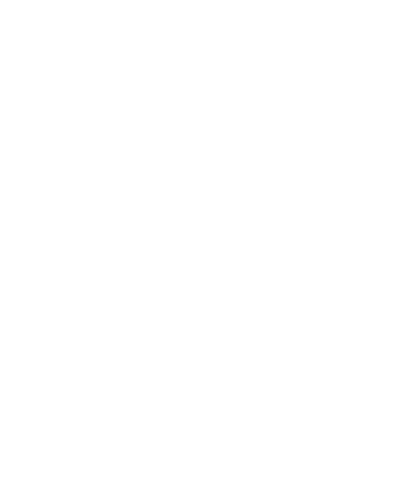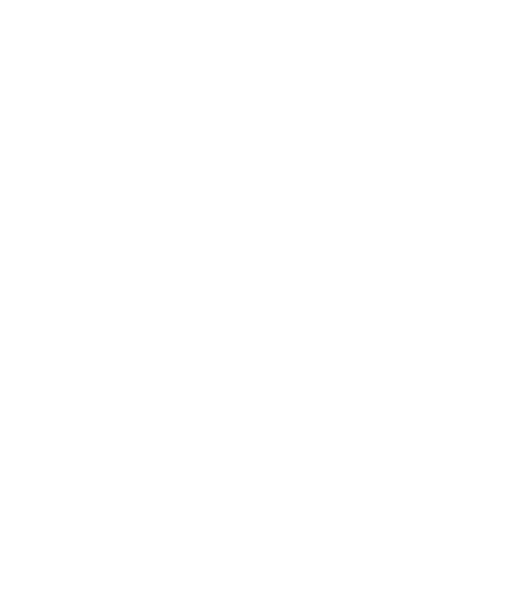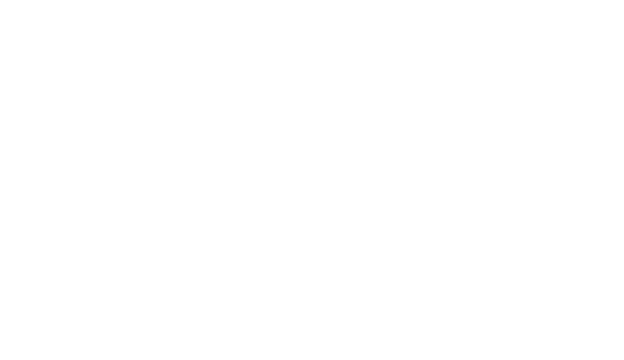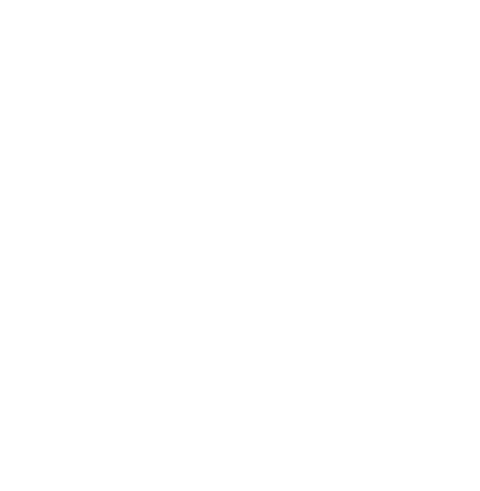# Basic Image Processing Using NumPy and OpenCV

0
1148## IntroductionBasic Image Processing Using NumPy

In this article, you will learn Basic Image Processing Using NumPy and OpenCV like how to blur the image. Nowadays most of the smartphones have a Blur feature. What is means blur? It changes the image pixel of a particular region, generally change the pixel of that region there is no object.

Image blur also called Image Smoothing.

Image blurring is achieved by convolving the image with a low-pass filter kernel. It is useful for removing noise. It actually removes high-frequency content (eg: noise, edges) from the image. So edges are blurred a little bit in this operation (there are also blurring techniques which don’t blur the edges). OpenCV provides four main types of blurring techniques.

Now, this program above is using an image blurring technique called Averaging.

There are some other options available as well – Gaussian Blurring, Median Blurring, Bilateral Filtering.

Basic Image Processing Using NumPy and OpenCV include drawing polygon, background subtraction, image threshold etc.

## Drawing function in OpenCV

Below function, you will see some common arguments as given below:

• Img : The image where you want to draw the shapes
• Color : Color of the shape. for BGR, pass it as a tuple, eg: (255,0,0) for blue. For grayscale, just pass the scalar value.
• Thickness: Thickness of the line or circle etc. If -1 is passed for closed figures like circles, it will fill the shape. default thickness = 1
• LineType: Type of line, whether 8-connected, anti-aliased line, etc.
``````Draw Rectangle
To draw a rectangle, you need a top-left corner and bottom-right corner of the rectangle.

# Python3 program to draw a rectangle
# shape on the solid image
import numpy as np
import cv2

# Creating a black image with 3
# channels RGB and unsigned int datatype
img = np.zeros((400, 400, 3), dtype = "uint8")

# Creating rectangle
cv2.rectangle(img, (30, 30), (300, 200), (0, 255, 0), 5)
cv2.imshow('dark', img)
# Allows us to see image
# untill closed forcefully
cv2.waitKey(0)
cv2.destroyAllWindows()
``````## Draw Polygon

To draw a polygon, first, you need coordinates of vertices. Make those points into an array of shape ROWSx1x2 where ROWS are a number of vertices and it should be of type int32.

``````import numpy as np
import cv2

img = np.zeros((512, 512, 3), dtype = "uint8")
penta=np.array([[[40,160],[120,100],[200,160],[160,240],[80,240]]], np.int32)
triangle = np.array([[[240, 130], [380, 230], [190, 280]]], np.int32)
cv2.polylines(img, [triangle], True, (0,255,0), thickness=3)
img_mod = cv2.polylines(img, [penta], True, (255,120,255),3)
cv2.imshow('Shapes', img_mod)
cv2.waitKey(0)
cv2.destroyAllWindows()
``````NOTE: If the third argument is False, you will get polylines joining all the points, not a closed shape.

cv.polylines() can be used to draw multiple lines. Just create a list of all the lines you want to draw and pass it to the function. All lines will be drawn individually. It is a much better and faster way to draw a group of lines than calling cv.line() for each line.

## Background Subtraction Method

Background subtraction (BS) is a common and widely used technique for generating a foreground mask (namely, a binary image containing the pixels belonging to moving objects in the scene) by using static cameras.

As the name suggests, BS calculates the foreground mask performing a subtraction between the current frame and a background model, containing the static part of the scene or, more in general, everything that can be considered as background given the characteristics of the observed scene.

• Background modeling consists of two main steps:
1. Background Initialization;
2. Background Update.

In the first step, an initial model of the background is computed, while in the second step that model is updated in order to adapt to possible changes in the scene.

``````Import required Library
import cv2
import numpy as np

gray_img = cv2.cvtColor(img, cv2.COLOR_BGR2GRAY)
_, thresh = cv2.threshold(gray_img, 127, 255, cv2.THRESH_BINARY_INV + cv2.THRESH_OTSU)
``````
``````Image Contours
img_contours= cv2.findContours(thresh, cv2.RETR_TREE, cv2.CHAIN_APPROX_SIMPLE)[-2]

#Sort the contours:
img_contours=sorted(img_contours, key=cv2.contourArea)

for i in img_contours:

if cv2.contourArea(i) > 100:

break

cv2.drawContours(mask, [i],-1, 255, -1) #draw countr

#the bitwise_and operator

#display original image
cv2.imshow("Original Image", img)

#resultant image
cv2.imshow("Image with background removed", new_img)

cv2.waitKey(0)

#put output

``````

## Image Thresholding

In this tutorial, you will learn Simple thresholding, Adaptive thresholding,

### Simple Thresholding

Here, the matter is straight forward. If the pixel value is greater than a threshold value, it is assigned one value (maybe white), else it is assigned another value (maybe black). The function used is ‘cv2.threshold’. The first argument is the source image, which should be a grayscale image. The second argument is the threshold value which is used to classify the pixel values. The third argument is the maxVal which represents the value to be given if the pixel value is more than (sometimes less than) the threshold value. OpenCV provides different styles of thresholding and it is decided by the fourth parameter of the function. Different types are:

• cv2.THRESH_BINARY
• cv2.THRESH_BINARY_INV
• cv2.THRESH_TRUNC
• cv2.THRESH_TOZERO
• cv2.THRESH_TOZERO_INV
``````#importing the required libraries
import numpy as np
import cv2
import matplotlib.pyplot as plt
%matplotlib inline

#here 0 means that the image is loaded in gray scale format

ret,thresh_binary = cv2.threshold(gray_image,127,255,cv2.THRESH_BINARY)
ret,thresh_binary_inv=cv2.threshold(gray_image,127,255,cv2.THRESH_BINARY_INV)

ret,thresh_trunc=cv2.threshold(gray_image,127,255,cv2.THRESH_TRUNC)

ret,thresh_tozero=cv2.threshold(gray_image,127,255,cv2.THRESH_TOZERO)

ret,thresh_tozero_inv=cv2.threshold(gray_image,127,255,cv2.THRESH_TOZERO_INV)

#DISPLAYING THE DIFFERENT THRESHOLDING STYLES
names=['Oiriginal Image','BINARY','THRESH_BINARY_INV','THRESH_TRUNC','THRESH_TOZERO','THRESH_TOZERO_INV']

images=gray_image,thresh_binary,thresh_binary_inv,thresh_trunc,thresh_tozero,thresh_tozero_inv

for i in range(6):
plt.subplot(2,3,i+1),plt.imshow(images[i],'gray')
plt.title(names[i])
plt.xticks([]),plt.yticks([])

plt.show()

``````In the previous section, we used a global value as the threshold value. But it may not be good in all the conditions where the image has different lighting conditions in different areas. In that case, we go for adaptive thresholding. In this, the algorithm calculates the threshold for small regions of the image. So we get different thresholds for different regions of the same image and it gives us better results for images with varying illumination.

It has three ‘special’ input params and only one output argument.

• Adaptive Method – It decides how thresholding value is calculated.

cv2.ADAPTIVE_THRESH_MEAN_C: threshold value is the mean of the neighbourhood area.

cv2.ADAPTIVE_THRESH_GAUSSIAN_C: threshold value is the weighted sum of neighborhood values where weights are a gaussian window.

Block Size – It decides the size of the neighborhood area.

C – It is just a constant which is subtracted from the mean or weighted mean calculated.

Below piece of code compares global thresholding and adaptive thresholding for an image with varying illumination:

``````import cv2
import numpy as np
from matplotlib import pyplot as plt

img = cv2.medianBlur(img,5)

ret,th1=cv2.threshold(img,127,255,cv2.THRESH_BINARY)

titles = ['Original Image', 'Global Thresholding (v = 127)',

images = [img, th1, th2, th3]

for i in xrange(4):
plt.subplot(2,2,i+1),plt.imshow(images[i],'gray')
plt.title(titles[i])
plt.xticks([]),plt.yticks([])
plt.show()
``````## Conclusion

In this article, we understand about blur the image with types and how it works. The next important step is Histogram equalization it can show the graph as per intensity.